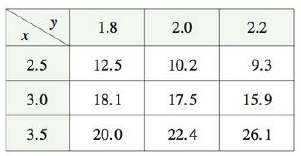Chapter 14.3, Problem 73E

Chapter
Section
Textbook Problem

Use the table of values of f(x, y) to estimate the values of fx(3, 2), fx(3, 2.2),and fxy(3, 2).To determine

To estimate: The values of fx(3,2) , fx(3,2.2) and fxy(3,2) by using the table of values of f(x,y) .

Explanation

Definition used:

If f is a function of two variables, its partial derivatives fx and fy are defined as a limit by,

fx(x,y)=limh0f(x+h,y)f(x,y)hfy(x,y)=limh0f(x,y+h)f(x,y)h

Calculation:

By definition, fx(3,2)=limh0f(3+h,2)f(3,2)h .

Assume h=0.5 and use the given Table to obtain the value of fx(3,2) .

fx(3,2)f(3+0.5,2)f(3,2)0.5f(3.5,2)f(3,2)0.5=22.417.50.5=4.90.5=9.8

Then, assume h=0.5 and use the given Table to obtain the values of fx(3,2) .

fx(3,2)f(30.5,2)f(3,2)0.5f(2.5,2)f(3,2)0.5=10.217.50.5=7.30.5=14.6

Obtain the average of above two values of fx(3,2) ,

fx(3,2)=9.8+14.62=24.42=12.2

Thus, the value of fx(3,2)=12.2 .

The partial derivative fxy(3,2) is defined as,

fxy(3,2)=y[fx(3,2)]=limh0fx(x,y+h)fx(x,y)h=limh0fx(3,2+h)fx(3,2)h

If h=0.2 , then fxy(3,2)=limh0fx(3,2.2)fx(3,2)h . (1)

If h=0.2 , then fxy(3,2)=limh0fx(3,1.8)fx(3,2)h . (2)

By definition, fx(3,2.2)=limh0f(3+h,2.2)f(3,2.2)h .

Assume h=0.5 and use the given Table to obtain the value of fx(3,2.2) .

fx(3,2.2)f(3+0.5,2.2)f(3,2

Still sussing out bartleby?

Check out a sample textbook solution.

See a sample solution

The Solution to Your Study Problems

Bartleby provides explanations to thousands of textbook problems written by our experts, many with advanced degrees!

Get Started

Solve the equations in Exercises 112 for x (mentally, if possible). x+1=3x+1

Finite Mathematics and Applied Calculus (MindTap Course List)

Define sampling with replacement and explain why is it used?

Statistics for The Behavioral Sciences (MindTap Course List)

In Exercises 2528. solve the equation by factoring. 28. 2x4 + x2 = 1

Applied Calculus for the Managerial, Life, and Social Sciences: A Brief Approach